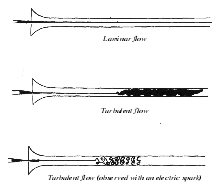I had an instance at work where I needed to quickly calculate an estimate for Reynolds Number for 0 gallons/min to 10 gallons/min for several different pipe diameters.

$$Re = \frac{QD}{vA}$$
• $$Re$$ = Reynolds Number (dimensionless)
• $$Q$$ = volumetric flow rate ($$m^3/s$$)
• $$v$$ = kinematic viscocity of the fluid ($$m^2/s$$)
• $$A$$ = cross sectional area ($$m^2$$)

At first, I tried this by hand. With various unit conversions, I got something wrong and ended up off by about 50%. Enter Python and Pint.

You probably already know about Python, but Pint is a very handy little package that keeps track of your units as you are going through, including through math operations. This keeps you from messing things up due to a simple unit conversion error.

# The Code

First, we set ourselves up:

import pint
from math import pi

unit = pint.UnitRegistry()  # create the unit registry


We then define our central calculation, implementing the formula above. Note the .to() method that is being utilized to get the units consistent. Pint will often resolve simple operations itself - unit.inch * unit.meter will result in inch ** 2 - but Pint is not a symbolic math engine, it just cancels the appropriate units that are obvious and leaves the rest out there. As a result, your answer may be technically correct, but useless with mixed units everywhere if you don't use the .to() method.

def calc_reynolds_number(volumetric_flow_rate, hydraulic_diameter, kinematic_viscocity, cross_sectional_area):
q = volumetric_flow_rate.to('meter ** 3 / s')
dh = hydraulic_diameter.to('meter')
v = kinematic_viscocity.to('meter ** 2 / second')
a = cross_sectional_area.to('meter ** 2')

re = (q * dh) / (v * a)

return re


As it turns out, the presented example results in the same exact output if the function were re-written:

def calc_reynolds_number(volumetric_flow_rate, hydraulic_diameter, kinematic_viscocity, cross_sectional_area):
re = (volumetric_flow_rate * hydraulic_diameter) / (kinematic_viscocity * cross_sectional_area)
return re


So there's one point for Pint. I have had the non-conversion bite me in the past, so I always convert to my preferred units.

Finally, we need to actually perform our calculations and show the results:

if __name__ == '__main__':
viscosity = 0.000001 * unit.meter ** 2 / unit.second
cross_sectional_area = pi * (0.5 * unit.inch) ** 2
diameter = 2.0 * unit.inch

for i in range(11):
volumetric_flow_rate = i * unit.gallons / unit.minute
re = calc_reynolds_number(volumetric_flow_rate, diameter, viscosity, cross_sectional_area)

print('{}\t{}'.format(volumetric_flow_rate, int(re)))


This results in an output of:

0.0 gallon / minute 0
1.0 gallon / minute 6325
2.0 gallon / minute 12650
3.0 gallon / minute 18975
4.0 gallon / minute 25300
5.0 gallon / minute 31625
6.0 gallon / minute 37950
7.0 gallon / minute 44275
8.0 gallon / minute 50600
9.0 gallon / minute 56926
10.0 gallon / minute    63251


Which I can easily copy/paste into my favorite spreadsheet editor!

© by Jason R. Jones 2016
My thanks to the Pelican and Python Communities.